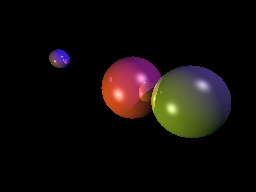# Project 5: Rendering reflections

## Goals

• Recursively apply the ray tracing algorithm to render reflections

In this project, we will cast a ray from each intersection point at an angle equal to the angle of incidence. This will allow us to see what that intersection point is "seeing", meaning we will be performing the ray tracing algorithm recursively.Each of the three spheres shows a reflected image of at least one of the other spheres. Notice the reflections are tinted to the color of the sphere on which the reflection appears.

## Model the reflectivity of a material

In each of the materials in the scene, add a new constant, k_r, representing the reflectivity of a material. This constant, like the other material constants, is a color, so the material reflects different amounts for each of the red, green and blue components.

If you set a sphere's reflectivity to have a high component, reduce that sphere's diffuse constant for that same component. This simulates an object being more reflective, and thus scattering light in fewer directions.

## Set up a recursion limit

In preparation to call the ray tracing algorithm recursively, we need to ensure the entire algorithm is packaged up as a function. The function simply takes a ray and the scene, then returns a color stating what the corresponding pixel should be colored. You may have already written your algorithm this way.

Once the algorithm is available as a function, include one more parameter. This parameter is simply the current recursion depth, and we will not call the algorithm recursively once the maximum depth is reached. A good depth is three bounces.

## Calculate the reflectance vectorThis shows a light vector being reflected across the surface normal. For calculating reflections, we care about the view vector, hat bbV, described below, instead of the light vector.

Each time a ray intersects a sphere, calculate the reflectance vector. Start by taking the direction of the incoming ray, then reverse and normalize it. To reverse a vector, multiply it by -1. This is hat bbV. So far, the incoming ray is just the ray we cast from the camera, but once we start applying the ray tracing algorithm recursively, this will be whatever ray was cast in the previous step.

Now, calculate the reflectance vector as hat bbR = 2(hat bbN * hat bbV)hat bbN - hat bbV.

All of this is only necessary if the maximum recursion depth has not been reached.

## Recursively apply the ray tracing algorithm

Create a new ray, with the origin being the point of intersection with the previous ray, and the direction being the reflectance vector. Call the ray tracing algorithm using this new ray, decreasing the recursion depth by one.

Once a color is returned from this recursive call, multiply it by k_r and add it to the color calculated by the Phong illumination model. Do this regardless of whether the intersection point is in shadow or not.

In the reference image, we can see clear reflections on the spheres: